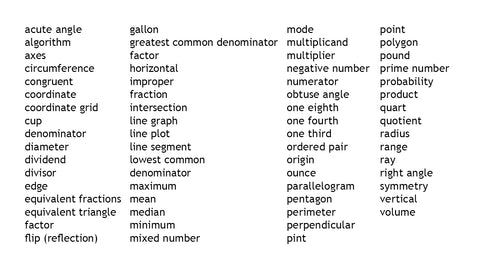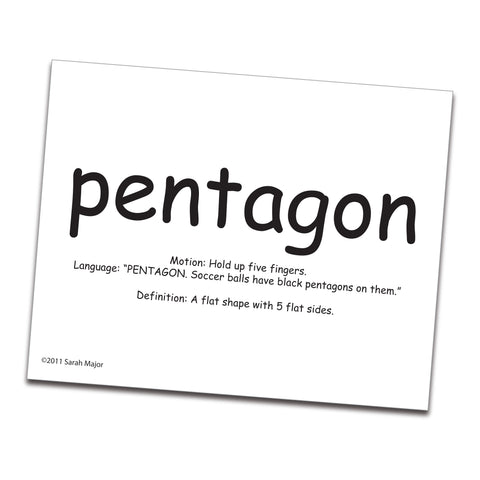# SnapWords® Math Vocabulary Set 3

SWMV3

Set 3 of SnapWords® Math Vocabulary picks up where Sets 1 and 2 left off. The more advanced the math concepts, such as fractions and graphing, the more abstract the math terms become. Here's where having images that show the meanings of the words saves the day!

SnapWords® Math Vocabulary cards make learning math language easier, and they help students understand the meaning behind each word. Sets 1-3 are a collection of 189 math terms in these categories:

• Location
• Geometry
• Calendar
• Classification
• Money
• Number/computation
• Measurement
• Fractions
• Graphing

#### Words Included in Set 3:On the front of each card, words are stylized to show their meaning visually, making it easy for students to learn and remember each word.On the back of each card you will find:

• a related body motion
• the term in a sentence
• a definition of the term